# 基尔霍夫电路定律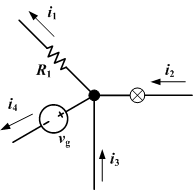$\sum_{k=1}^n i_k =0$

### [编辑]导引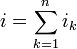$i=\sum_{k=1}^n i_k$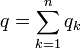$q=\sum_{k=1}^n q_k$$\sum_{k=1}^n i_k =0$

### [编辑]含时电荷密度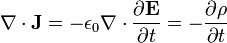$\nabla \cdot \mathbf{J} = -\epsilon_0\nabla \cdot \frac{\partial \mathbf{E}}{\partial t} = -\frac{\partial \rho}{\partial t}$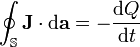$\oint_{\mathbb{S}}\mathbf{J}\cdot \mathrm{d}\mathbf{a} = -\frac{ \mathrm{d} Q}{ \mathrm{d} t}$

##### Tags：基尔霍夫,电路定律

• 上一个文章：
• 下一个文章：
• 相关文章列表
欧姆定律
请文明参与讨论，禁止漫骂攻击，不要恶意评论、违禁词语。 昵称：
 1分 2分 3分 4分 5分 还可以输入 200 个字
网友评论
推荐文章
最新推荐
热门文章